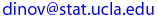Ivo Dinov
UCLA Statistics, Neurology, LONI
, Math/PIC
 Courses SOCR Ivo Dinov's Home SiteMap Software Contact

STAT 35
Winter  2005

Interactive and Computational Probability

Department of Statistics

## Instructor: Ivo Dinov

Homework 3
Due Date: Wednesday, Feb. 16 2005

Please, submit your homework right after lecture on the due date. See the HW submission rules. On the front page include the following header.

• (HW_3_1) Shortly after being put into service, some buses manufactured by a certain company have developed cracks on the underside of the main frame. Suppose a particular city has 20 of these buses, and cracks have actually appeared in 8 of them.
• How many ways are there to select a sample of 5 buses from the 20 for a thorough inspection?
• In how many ways can a sample of 5 buses contain exactly 4 with visible cracks?
• If a sample of 5 buses is chosen at random, what is the probability that exactly 4 of the 5 will have visible cracks?
• If buses are selected as in part (c), what is the probability that at least 4 of those selected will have visible cracks?

• (HW_3_2)  Three molecules of type A, 3 of type B, 3 of type C, and 3 of type D are to be linked together to form a chain molecule. One such chain molecule is ABCDABCDABCD, and another is BCDDAAABDBCC.
• How many such chain molecules are there?
• Suppose a chain molecule of the type desired is randomly selected. What is the probability that all three molecules of each type end up next to one another (such as BBBAAADDDCCC)?

• (HW_3_3) A certain sports car comes equipped with either an automatic or a manual transmission, and the car is available in one of four colors. Relevant probabilities for various combinations of transmission type and color are given in the table below. Let A = {automatic transmission} , B = { black } , and C = { white }.  Color White Blue Black Red Transmission Type A 0.15 0.10 0.10 0.10 M 0.15 0.05 0.15 0.20

(a) Calculate P(A), P(B), and P(A ∩ B).
(b) Calculate both P(A | B) and P(B | A), and explain in context what each of these probabilities represent.
(c) Calculate and interpret P(A | C) and P(A | C').

• (HW_3_4) Use the  Die Coin Experiments (http://socr.stat.ucla.edu) to emperically validate the Baysian rule for computing conditional probabilities:
• Suppose D3 = event { Die turns up a three (first part of the experiment) } and 2H = event { There are two Heads observed in the tossed coins (second part of the experiment) }. Run the experiment 100 times first with flat-die-probabilities (p=1/6) and a fair-coin (p=0.5). By counting outcomes of interest validate computationally that:
• P( D3 | 2H ) = [ P( 2H | D3 ) P( D3 ) ] / P( 2H )
• Now run the experiment 100 times first with a loaded die with probabilities (p1= 0.01, p2=0.05, p3=0.1, p4=0.2, p5=0.34, p6=0.3) and a loaded coin (p=0.3). Again by counting outcomes of interest validate computationally that:
• P( D3 | 2H ) = [ P( 2H | D3 ) P( D3 ) ] / P( 2H )

 Ivo Dinov's Home http://www.stat.ucla.edu/~dinov Visitor number, since Jan. 01, 2002 © I.D.Dinov 1997-2005
Last modified on by